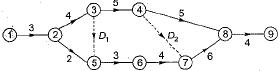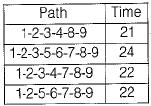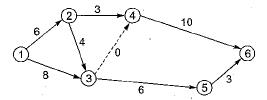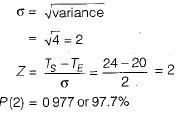Courses

# PERT And CPM - 1

## 10 Questions MCQ Test Topicwise Question Bank for GATE Mechanical Engineering | PERT And CPM - 1

Description
This mock test of PERT And CPM - 1 for Mechanical Engineering helps you for every Mechanical Engineering entrance exam. This contains 10 Multiple Choice Questions for Mechanical Engineering PERT And CPM - 1 (mcq) to study with solutions a complete question bank. The solved questions answers in this PERT And CPM - 1 quiz give you a good mix of easy questions and tough questions. Mechanical Engineering students definitely take this PERT And CPM - 1 exercise for a better result in the exam. You can find other PERT And CPM - 1 extra questions, long questions & short questions for Mechanical Engineering on EduRev as well by searching above.
QUESTION: 1

### In the network diagram shown below:The critical path is along

Solution:The critical path is along 1 -2-3-5-6-7-8-9 as it has maximum duration.

QUESTION: 2

### Refer to the network shown in the figure given belowThe probability of completion of the project in 24 days is

Solution:

The critical path is 1-2-3-4-6.
Ts - Scheduled time
= 24 days
Te - Expected time = 20 daysQUESTION: 3

### The activities for which total float is equal to zero are known as

Solution:
QUESTION: 4

The time by which as activity can be delayed without affecting the earliest start time of any other activity in a project is called

Solution:
QUESTION: 5

The slack on various events at critical path on a PERT/ CPM chart

Solution:
QUESTION: 6

Which one of the following is assumed for timing the activities in PERT network?

Solution:
QUESTION: 7

The area under β-distribution curve is divided into two equal halves by a vertical oridnate through

Solution:
QUESTION: 8

Negative slack occurs when

Solution:
QUESTION: 9

Which of the following is not a logical error in network construction?

Solution:
QUESTION: 10

The performance of a specific task in CPM is known as

Solution: